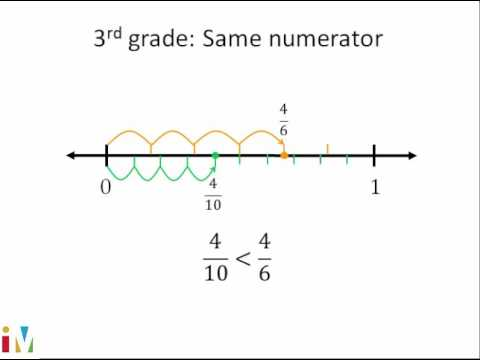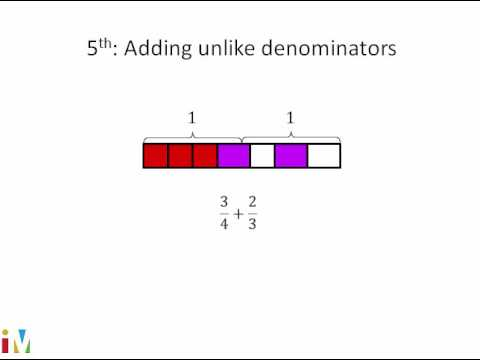Video

# Comparing fractions 2 (unlike denominators) (Full video)

Description: Sal compares fractions by finding a common denominator. Use less than, greater than, or equal to compare the two fractions 21/28, or 21 over 28, and 6/9, or 6 over 9. The easiest way is if they had the same denominator, you could just compare the numerators. So what we could do is we can find a common denominator for both of them and convert both of these fractions to have the same denominator and then compare the numerators.

### Other videos you might be interested in### Comparing Fractions

#### Illustrative Mathematics